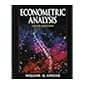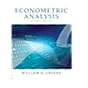Normal view MARC view ISBD view

# Basic econometrics Damodar N. Gujarati, Dawn C. Porter.

Material type:TextPublisher: Boston : McGraw-Hill, 2009; New Delhi, Tata McGraw Hill Education Private ltd. 2012Edition: 5th ed.Description: xxiii, 886 p. : ill. ; 24 cm.ISBN: 9780071276252; 0071276254; 9780071333450; 0071333452.DDC classification: 330.015195 GUB Online resources: WorldCat details | E-book Fulltext
Contents:
Introduction -- Nature of regression analysis -- Two-variable regression analysis : some basic ideas -- Two-variable regression model: the problem of estimation -- Classical normal linear regression model -- Two-variable regression: interval estimation and hypothesis testing -- Extensions of the two-variable linear regression model -- Multiple regression analysis : the problem of estimation -- Multiple regression analysis : the problem of inference -- Dummy variable regression model -- Multicollinearity : what happens in the regressors are correlated? -- Heteroscedasticity: what happens if the error variance is nonconstant? -- Autocorrelation: what happens if the error terms are correlated? -- Economic modeling: model specification and diagnostic testing -- Nonlinear regression models -- Qualitative response regression models -- Panel data regression models -- Dynamic econometric models : autoregressive and distributed-lag models -- Simultaneous-equation models -- Identification problem -- Simultaneous-equation methods -- Time series econometrics : some basic concepts -- Time series econometrics : forecasting -- Appendix A : a review of some statistical concepts -- Appendix B : rudiments of matrix algebra -- Appendix C : matrix approach to linear regression model -- Appendix D : statistical tables -- Appendix E : computer output of EViews, MINITAB, Excel, and STATA -- Appendix F : economic data on the World Wide Web.
Tags from this library: No tags from this library for this title.Average rating: 0.0 (0 votes)
Item type Current location Collection Call number Copy number Status Date due Barcode Item holdsE-Book
E-book
Non-fiction 330.015195 GUB 2009 (Browse shelf) Not For LoanText
Reserve Section
Non-fiction 330.015195 GUB 2009 (Browse shelf) C-1 Not For Loan 25590Text
Reserve Section
Non-fiction 330.015195 GUB 2009 (Browse shelf) C-2 Available 25591Text
Circulation Section
Non-fiction 330.015195 GUB 2009 (Browse shelf) C-3 Checked out 28/08/2019 25592
Total holds: 0
##### Browsing EWU Library Shelves , Shelving location: E-book Close shelf browser330.015195 GRE 2008 Econometric analysis / 330.015195 GRE 2012 Econometric analysis / 330.015195 GUB 2003 Basic econometrics / 330.015195 GUB 2009 Basic econometrics 330.015195 HSA 2014 Analysis of panel data / 330.015195 JOE 1997 Econometric methods / 330.015195 JUN 1998 Numerical methods in economics /

Includes bibliographical references (p. 902-903) and indexes.

Introduction --
Nature of regression analysis --
Two-variable regression analysis : some basic ideas --
Two-variable regression model: the problem of estimation --
Classical normal linear regression model --
Two-variable regression: interval estimation and hypothesis testing --
Extensions of the two-variable linear regression model --
Multiple regression analysis : the problem of estimation --
Multiple regression analysis : the problem of inference --
Dummy variable regression model --
Multicollinearity : what happens in the regressors are correlated? --
Heteroscedasticity: what happens if the error variance is nonconstant? --
Autocorrelation: what happens if the error terms are correlated? --
Economic modeling: model specification and diagnostic testing --
Nonlinear regression models --
Qualitative response regression models --
Panel data regression models --
Dynamic econometric models : autoregressive and distributed-lag models --
Simultaneous-equation models --
Identification problem --
Simultaneous-equation methods --
Time series econometrics : some basic concepts --
Time series econometrics : forecasting --
Appendix A : a review of some statistical concepts --
Appendix B : rudiments of matrix algebra --
Appendix C : matrix approach to linear regression model --
Appendix D : statistical tables --
Appendix E : computer output of EViews, MINITAB, Excel, and STATA --
Appendix F : economic data on the World Wide Web.

Computer Science & Engineering

There are no comments for this item.